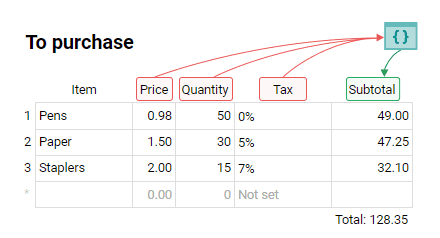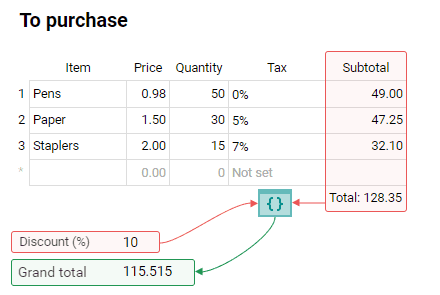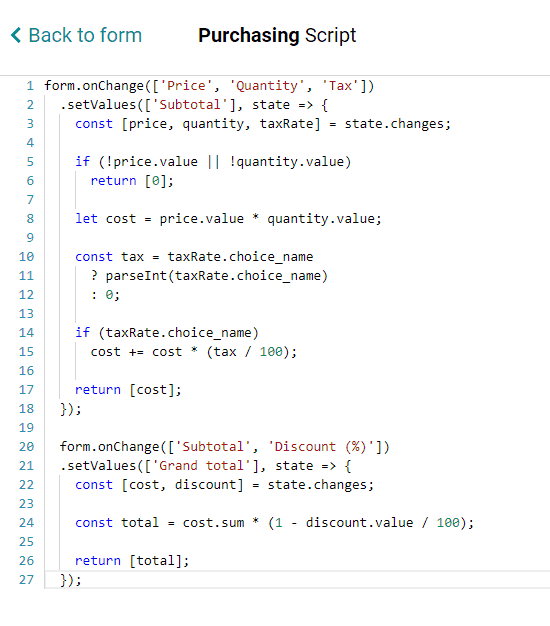# Tables

Scripts can be used in forms that contain tables. Use them to:

• calculate table field values based on the values of cells in the same row.
• reference the overall sum of the values in a table column to calculate the value of a non-table field.

Unlike automatically calculated table fields, scripts allow you to define conditions and support other field types, like multiple choice.

### Creating a calculable table field

Suppose you have a form that contains a table with a list of items available for purchase. Each item has a Name, Price, Quantity, Subtotal, and Tax field. Tax is a multiple choice field with three options: 0%, 5% and 7%.The value of the Subtotal field can be automatically calculated and filled in with help from this piece of code:

```form.onChange(['Price', 'Quantity', 'Tax'])
.setValue('Subtotal', state => {
const [price, quantity, taxRate] = state.changes;

if (!price || !price.value || !quantity || !quantity.value || !taxRate)
return 0;

let cost = price.value * quantity.value;

const tax = taxRate.choice_name
? parseInt(taxRate.choice_name)
: 0;

if (taxRate.choice_name)
cost += cost * (tax / 100);

return cost;
});
```

### Creating a calculable column

Let’s place two fields, Discount and Grand total, at the bottom of the table.To automatically calculate the Grand total (the total after the discount), use this code:

```form.onChange(['Subtotal', 'Discount'])
.setValue('Grand total', state => {
const [cost, discount] = state.changes;

if (!cost || !discount)
return null;

const total = cost.sum * (1 - discount.value / 100);